# Modules Grouped by General Applied Topic

### [ Regrouping by General Mathematics Topic ]

 ready 1.00.80.60.40.20.0 not ready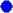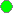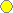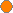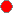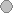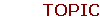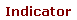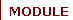Mechanical OscillationsVibrating Strings Forced Spring Mass Spring Mass Fourier Series Linear Pendulum Spring Pendulum Nonlinear Pendulum Multiple Spring Mass System Probability and StatisticsMeans and Variances Continuous Random Variables Inventory Control Conditional Probability Poisson and Exponential Distributions Random Variable Relations Electricity and MagnetismElectric Potential Electric Field Gauss's Law Faraday's Law and Induction Resistance & Capacitance Electromagnetic Oscillations Ampere's Law Transport PhenomenaDrag Forces on Solid Objects Mass Transport The Gradient Lake Pollution Sequential Batch Reactions Heat Conduction I Heat Conduction II Chemical Kinetics and Equilibria Continuously Stirred Tank Reactor Flux and Surface Integration Linear SystemsBicycle Matrix Kit Crystallography System DesignConstrained Optimization Curvature and Curve Design Boundary Value Problems for ODEs Graph TheoryIndustrial Drilling Networking Sperner's Lemma Graph Isomorphismhome